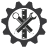SuperTeacherTools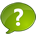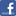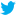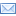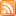### 6th Grade Math Jeopardy Speed Match Review Game

This game has been played 3 times
The best time ever was piglet at 377 seconds.
The best time today is at seconds.
How To Play:
Drag the squares in the gray area and drop them in the matching squares in the blue area below.
If you drop a square in the correct spot, it will disappear.
If you make a mistake, the square will return to its original spot.
Good luck!

Let's Play Speed Match!

The answer is:  45

Answer:

84 - 1,2,3,4,6,7,12,14,21,28,42,84

120 - 1,2,3,4,5,6,8,10,12,15,20,24,30,40,60,120

GCF is 12

Answer:

A = 337.5 cm

Answer:

Area is 180 yd2

Answer is:  9.92

The answer is:

1/2

The answer is:

61.1

The Answer is:  126

The Answer is:

6 39/60

The answer is:

4 4/5

Answer:

Look for the GCF

18 - 1,2,3,6,9,18

24 - 1,2,3,4,6,8,12,24

The GCF is 6.  Therefore the greatest number of students that can be in a row is 6.

The Answer is:

1,2,4,8,16,32,64

The answer is 69

The answer is:  83

The answer is:  35.4

Answer:  31.4 in

The Answer is:

16/35

Answer:

The student did not subtract 12 - 3 before squaring.  It should look like this:

2 × (12-3)2 + 7

2 × 92 + 7

2 × 81 + 7

162 + 7

169

Answer:

7.5 in22

The answer is:

7.5

Answer:

16, 32, 48, 64, 80

Answer: 74 m

The answer is:  2.940

Answer:

5- 5,10,15,20,25,30,35,40,45,50

6, - 6,12,18,24,30,36,42,48

10 - 10,20,30,40,50

LCM is 30

The Answer is:

7/40

What is the GCF of 84 and 120?

Evaluate the expression:

40.4 - 5

Evaluate the expression:  100 - 80 ÷ 4 + 3

Here is how a student simplified an expression.  Is this correct? If not, where did the student amek a mistake?

2 × (12-3)2 +7

2 × (12-9) + 7

2 × 3 + 7

6 + 7

13

Evaluate the Expression:

7/8 ÷ 5

Find the circumference of a circle with a radius of 5 in.

C = 2∏ r

Evaluate the expression:

281.06 ÷ 4.6

Evaluate the following expression:

7.68 + 2.24

Evaluate the Expression:

2 1/10 × 3 1/6

What is the LCM of 5, 6, and 10?

List all the factors of 64

Evaluate the expression:

5 1/2 - 7/10

Evaluate the expression:

8.4 × 0.35

Evaluate the Expression:

2/5 ÷ 7/8

What is the area of a a Parallelogram with base of 15 yd and a height of 12 yd?

Evaluate the expression:  6 + 7 × 9

Evaluate the expression: 52 × 4 ÷ 2 - (8-3)

What is the area of a triangle witha base of 5 in and a heigth of 3 in?

A =  1/2 bh

List the first five Multiples of 16.

Evaluate the expression:

6.00 ÷ 0.8

Evaluate the express :  (8+3)2 - 3 + 8

In a parade, 18 boys and 24 girls are merching in seperate rows.  Each rwo requires the same number of students.  What is the greatest number of students that can be in a row?

Evaluate the expression:

3/10 + 1/5

Find the area of a triangle owith heigth of 27 cm and a base of 25 cm.

A = 1/2 bh

What is the perimeter of a rectangle with width of 25 m and a heigth of 12 m?

## You did it!What is your name?

## Here are all the answers:

DescriptionMatch:

Evaluate the express :  (8+3)2 - 3 + 8

The Answer is:  126

Evaluate the expression:  100 - 80 ÷ 4 + 3

The answer is:  83

Evaluate the expression:  6 + 7 × 9

The answer is 69

Evaluate the expression: 52 × 4 ÷ 2 - (8-3)

The answer is:  45

Here is how a student simplified an expression.  Is this correct? If not, where did the student amek a mistake?

2 × (12-3)2 +7

2 × (12-9) + 7

2 × 3 + 7

6 + 7

13

Answer:

The student did not subtract 12 - 3 before squaring.  It should look like this:

2 × (12-3)2 + 7

2 × 92 + 7

2 × 81 + 7

162 + 7

169

Evaluate the following expression:

7.68 + 2.24

Answer is:  9.92

Evaluate the expression:

40.4 - 5

The answer is:  35.4

Evaluate the expression:

8.4 × 0.35

The answer is:  2.940

Evaluate the expression:

6.00 ÷ 0.8

The answer is:

7.5

Evaluate the expression:

281.06 ÷ 4.6

The answer is:

61.1

Evaluate the expression:

3/10 + 1/5

The answer is:

1/2

Evaluate the expression:

5 1/2 - 7/10

The answer is:

4 4/5

Evaluate the Expression:

2 1/10 × 3 1/6

The Answer is:

6 39/60

Evaluate the Expression:

2/5 ÷ 7/8

The Answer is:

16/35

Evaluate the Expression:

7/8 ÷ 5

The Answer is:

7/40

List all the factors of 64

The Answer is:

1,2,4,8,16,32,64

List the first five Multiples of 16.

Answer:

16, 32, 48, 64, 80

What is the GCF of 84 and 120?

Answer:

84 - 1,2,3,4,6,7,12,14,21,28,42,84

120 - 1,2,3,4,5,6,8,10,12,15,20,24,30,40,60,120

GCF is 12

What is the LCM of 5, 6, and 10?

Answer:

5- 5,10,15,20,25,30,35,40,45,50

6, - 6,12,18,24,30,36,42,48

10 - 10,20,30,40,50

LCM is 30

In a parade, 18 boys and 24 girls are merching in seperate rows.  Each rwo requires the same number of students.  What is the greatest number of students that can be in a row?

Answer:

Look for the GCF

18 - 1,2,3,6,9,18

24 - 1,2,3,4,6,8,12,24

The GCF is 6.  Therefore the greatest number of students that can be in a row is 6.

What is the perimeter of a rectangle with width of 25 m and a heigth of 12 m?

Answer: 74 m

What is the area of a a Parallelogram with base of 15 yd and a height of 12 yd?

Answer:

Area is 180 yd2

What is the area of a triangle witha base of 5 in and a heigth of 3 in?

A =  1/2 bh

Answer:

7.5 in22

Find the area of a triangle owith heigth of 27 cm and a base of 25 cm.

A = 1/2 bh

Answer:

A = 337.5 cm

Find the circumference of a circle with a radius of 5 in.

C = 2∏ r

Answer:  31.4 in